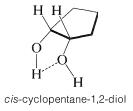# 15.3: Spectroscopic Properties of Alcohols

$$\newcommand{\vecs}{\overset { \rightharpoonup} {\mathbf{#1}} }$$ $$\newcommand{\vecd}{\overset{-\!-\!\rightharpoonup}{\vphantom{a}\smash {#1}}}$$$$\newcommand{\id}{\mathrm{id}}$$ $$\newcommand{\Span}{\mathrm{span}}$$ $$\newcommand{\kernel}{\mathrm{null}\,}$$ $$\newcommand{\range}{\mathrm{range}\,}$$ $$\newcommand{\RealPart}{\mathrm{Re}}$$ $$\newcommand{\ImaginaryPart}{\mathrm{Im}}$$ $$\newcommand{\Argument}{\mathrm{Arg}}$$ $$\newcommand{\norm}{\| #1 \|}$$ $$\newcommand{\inner}{\langle #1, #2 \rangle}$$ $$\newcommand{\Span}{\mathrm{span}}$$ $$\newcommand{\id}{\mathrm{id}}$$ $$\newcommand{\Span}{\mathrm{span}}$$ $$\newcommand{\kernel}{\mathrm{null}\,}$$ $$\newcommand{\range}{\mathrm{range}\,}$$ $$\newcommand{\RealPart}{\mathrm{Re}}$$ $$\newcommand{\ImaginaryPart}{\mathrm{Im}}$$ $$\newcommand{\Argument}{\mathrm{Arg}}$$ $$\newcommand{\norm}{\| #1 \|}$$ $$\newcommand{\inner}{\langle #1, #2 \rangle}$$ $$\newcommand{\Span}{\mathrm{span}}$$$$\newcommand{\AA}{\unicode[.8,0]{x212B}}$$

The hydrogen-oxygen bond of a hydroxyl group gives a characteristic absorption band in the infrared but, as we may expect, this absorption is considerably influenced by hydrogen bonding. For example, in the vapor state (in which there is essentially no hydrogen bonding), ethanol gives an infrared spectrum with a fairly sharp absorption band at $$3700 \: \text{cm}^{-1}$$, owing to a free or unassociated hydroxyl group (Figure 15-2a). In contrast, this band is barely visible at $$3640 \: \text{cm}^{-1}$$ in the spectrum of a $$10\%$$ solution of ethanol in carbon tetrachloride (Figure 15-2b). However, there is a relatively broad band around $$3350 \: \text{cm}^{-1}$$, which is characteristic of hydrogen-bonded hydroxyl groups. The shift in frequency of about $$300 \: \text{cm}^{-1}$$ arises because hydrogen bonding weakens the $$\ce{O-H}$$ bond; its absorption frequency then will be lower. The association band is broad because the hydroxyl groups are associated in aggregates of various sizes and shapes. This produces a variety of different kinds of hydrogen bonds and therefore a spectrum of closely spaced $$\ce{O-H}$$ absorption frequencies.Figure 15-2: Infrared spectrum of ethanol (a) in the vapor phase and (b) as a $$10\%$$ solution in carbon tetrachloride.

In very dilute solutions of alcohols in nonpolar solvents, hydrogen bonding is minimized. However, as the concentration is increased, more and more of the molecules become associated and the intensity of the infrared absorption band due to associated hydroxyl groups increases at the expense of the free-hydroxyl band. Furthermore, the frequency of the association band is a measure of the strength of the hydrogen bond. The lower the frequency relative to the position of the free hydroxyl group, the stronger is the hydrogen bond. As we shall see in Chapter 18 the hydroxyl group in carboxylic acids $$\left( \ce{RCO_2H} \right)$$ forms stronger hydrogen bonds than alcohols and accordingly absorbs at lower frequencies (lower by about $$400 \: \text{cm}^{-1}$$, see Table 9-2).

The infrared spectra of certain 1,2-diols (glycols) are interesting in that they show absorption due to intramolecular hydrogen bonding. These usually are fairly sharp bands in the region $$3450 \: \text{cm}^{-1}$$ to $$3570 \: \text{cm}^{-1}$$, and, in contrast to bands due to intermolecular hydrogen bonding, they do not change in intensity with concentration. A typical example is afforded by cis-1,2-cyclopentanediol:Besides the $$\ce{O-H}$$ stretching vibrations of alcohols, there is a bending $$\ce{O-H}$$ vibration normally observed in the region $$1410$$-$$1260 \: \text{cm}^{-1}$$. There also is a strong $$\ce{C-O}$$ stretching vibration between $$1210 \: \text{cm}^{-1}$$ and $$1050 \: \text{cm}^{-1}$$. Both these bands are sensitive to structure as indicated below:The influence of hydrogen bonding on the proton nmr spectra of alcohols has been discussed previously (Section 9-10E). You may recall that the chemical shift of the $$\ce{OH}$$ proton is variable and depends on the extent of association through hydrogen bonding; generally, the stronger the association, the lower the field strength required to induce resonance. Alcohols also undergo intermolecular $$\ce{OH}$$ proton exchange, and the rate of this exchange can influence the line-shape of the $$\ce{OH}$$ resonance, the chemical shift, and the incidence of spin-spin splitting, as discussed in more detail in Sections 9-10E and 9-10I. Concerning the protons on carbon bearing the hydroxyl group, that is,, they are deshielded by the electron-attracting oxygen atom and accordingly have chemical shifts some $$2.5$$-$$3.0 \: \text{ppm}$$ to lower fields than alkyl protons.

Perhaps you are curious as to why absorptions are observed in the infrared spectrum of alcohols that correspond both to free and hydrogen-bonded hydroxyl groups, whereas only one $$\ce{OH}$$ resonance is observed in their proton nmr spectra. The explanation is that the lifetime of any molecule in either the free or the associated state is long enough to be detected by infrared absorption but much too short to be detected by nmr. Consequently, in the nmr one sees only the average $$\ce{OH}$$ resonance of the nonhydrogen-bonded and hydrogen-bonded species present. The situation here is very much like that observed for conformational equilibration (Section 9-10C).

The longest-wavelength ultraviolet absorption maxima of methanol and methoxymethane (dimethyl ether) are noted in Table 9-3. In each case the absorption maximum, which probably involves an $$n \rightarrow \sigma^*$$ transition, occurs about $$184 \: \text{nm}$$, well below the cut-off of the commonly available spectrometers.Figure 15-3: Proton nmr and infrared spectra (a) of $$\ce{C_4H_6O}$$ and (b) of $$\ce{C_3H_8O}$$.

The mass spectra of alcohols may not always show strong molecular ions. The reason is that the $$\ce{M^+}$$ ions readily fragment by $$\alpha$$ cleavage. The fragment ions are relatively stable and are the gaseous counterparts of protonated aldehydes and ketones:Ethers also fragment by $$\alpha$$ cleavage: